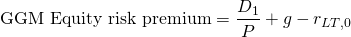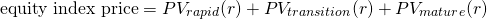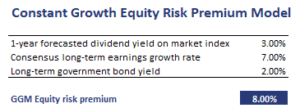# Gordon Equity Risk Premium Model

The Gordon Growth model (GGM) or constant growth model can be used to generate forward looking estimates of the equity risk premium (ERP). The GGM model is especially useful when applied to developed economies. That’s because reliable data on dividend payments and growth rates is generally easy to obtain.

We discuss the Gordon Growth model to estimate the equity risk premium, its strengths and weaknesses, and how it can be modified if an analyst wants to apply it to developing countries with different stages of growth. A numerical example in Excel is available at the bottom of the page.

The GGM estimates the risk premium as the expected dividend yield plus the expected growth rate minus the current long-term government bond yield. The formula equalswhere D/P is the 1-year forecasted dividend yield on the market index, g is the expected consensus long-term earnings growth rate, and r is the current long-term government bond yield.

## Weaknesses GGM Equity Risk Premium model

While the model is intuitively appealing, it has a number of weaknesses. First, forward-looking estimates may change over time. As a consequence, our forecasts of the equity-risk premium will be time-varying. Also, there is no guarantee that the capital appreciation will match the predicted value. A second weakness is the fact that the model assumes constant growth. While this is a reasonable assumption for developed economies, it generally does not hold true for rapidly growing economies. In these cases, growth may consist of a rapid growth, transition, and mature growth phase.

A better forward-looking estimate of the equity risk premium can be derived from the IRR from the following formulaFrom the resulting IRR we then need to subtract the government bond yield to obtain the equity risk premium.